# NPV Formula

Learn how Net Present Value works

## What is the NPV Formula?

The NPV formula is a way of calculating the Net Present Value (NPV) of a series of cash flows based on a specified discount rate.  The NPV formula can be very useful for financial analysis and financial modeling when determining the value of an investment (a company, a project, a cost-saving initiative, etc.).

Below is an illustration of the NPV formula for a single cash flow.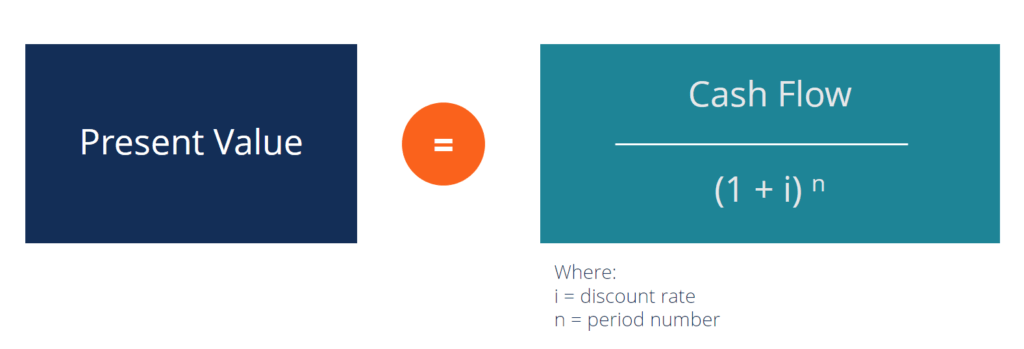Screenshot of CFI’s Corporate Finance 101 Course.

### NPV for a Series of Cash Flows

In most cases, a financial analyst needs to calculate the net present value of a series of cash flows, not just one individual cash flow.  The formula works in the same way, however, each cash flow has to be discounted individually, and then all of them are added together.

Here is an illustration of a series of cash flows being discounted: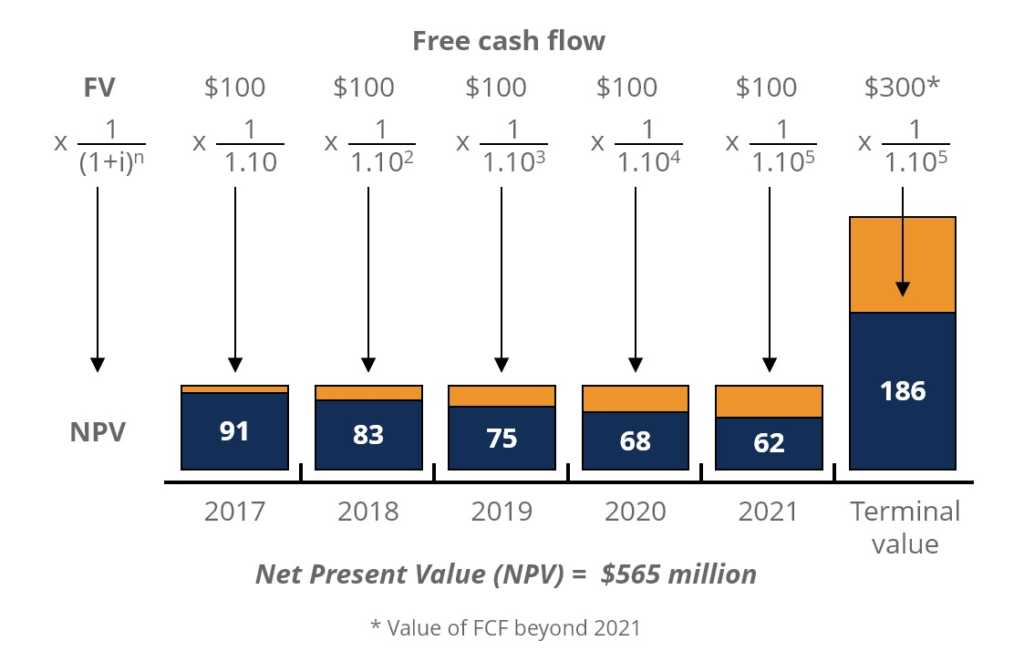Source: CFI’s Free Corporate Finance Course.

What is the Math Behind the NPV Formula?

Here is the mathematical formula for calculating the present value of an individual cash flow.

NPV = F / [ (1 + i)^n ]

Where,

PV = Present Value
F = Future payment (cash flow)
i = Discount rate (or interest rate)
n = the number of periods in the future the cash flow is

### How to Use the NPV Formula in Excel

Most financial analysts never calculate the net present value by hand nor with a calculator, instead, they use Excel.

=NPV(discount rate, series of cash flow)

(See screenshots below)

Example of how to use the NPV function:

Step 1: Set a discount rate in a cell.

Step 2: Establish a series of cash flows (must be in consecutive cells).

Step 3: Type “=NPV(“  and select the discount rate “,” then select the cash flow cells and “)”.

Congratulations, you have now calculated net present value in Excel!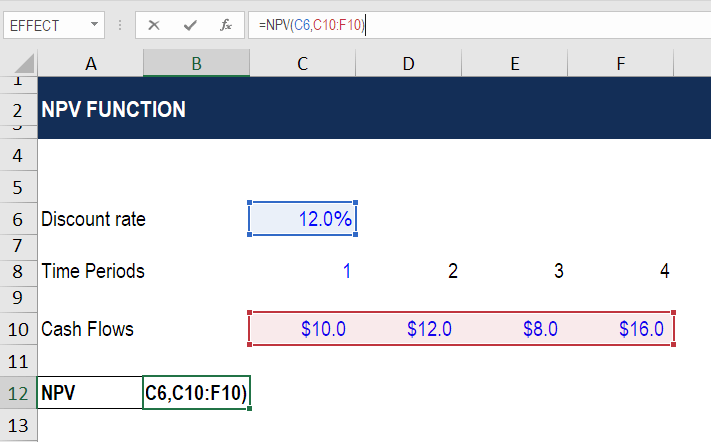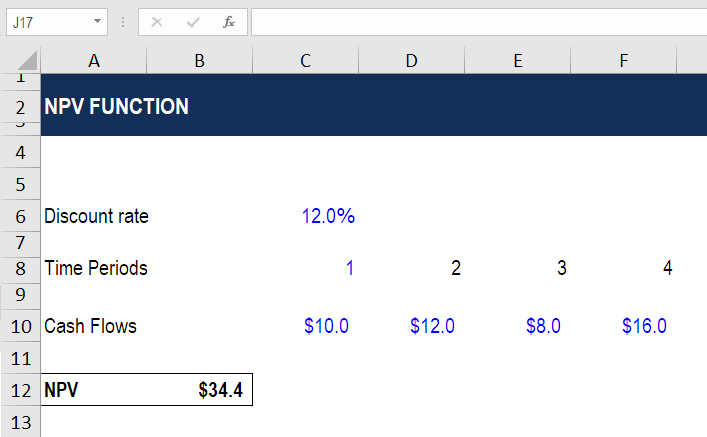Source: CFI’s Free Excel Crash Course.

If you need to be very precise in your calculation, it’s highly recommended to use XNPV instead of the regular function.

To find out why, read CFI’s guide to XNPV vs NPV in Excel.

### Video Explanation of the NPV Formula

Below is a short video explanation of how the formula works, including a detailed example with an illustration of how future cash flows become discounted back to the present.

### DCF Modeling

The main use of the NPV formula is in Discounted Cash Flow (DCF) modeling in Excel.  In DCF models an analyst will forecast a company’s three financial statements into the future and calculate the company’s Free Cash Flow to the Firm (FCFF). Additionally, a terminal value is calculated at the end of the forecast period. Each of the cash flows in the forecast and terminal value are then discounted back to the present using a hurdle rate of the firm’s weighted average cost of capital (WACC).

Below is an example of a DCF model from one of CFI’s financial modeling courses.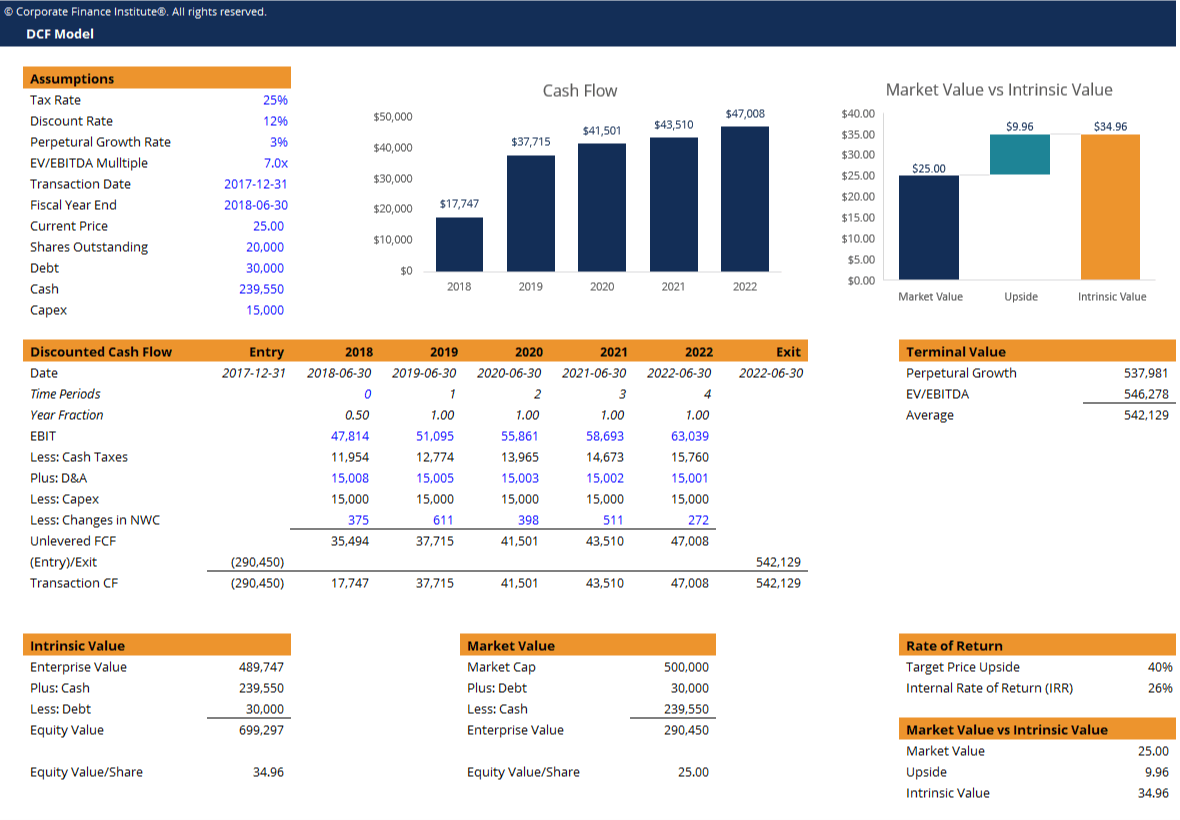Screenshot: CFI financial modeling courses.

Thank you for reading this guide to calculating net present value.  CFI’s mission is to help anyone become a world-class financial analyst.  To keep learning and advancing your career, these additional financial resources will be a big help:

• XNPV formula in Excel
• Walk me through a DCF model
• Valuation methods
• DCF modeling guide

### Valuation Techniques

Learn the most important valuation techniques in CFI’s Business Valuation course!

Step by step instruction on how the professionals on Wall Street value a company.

Learn valuation the easy way with templates and step by step instruction!